1.  Multiply the constants and then multiply the powers using the Power of a Product Property.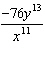2.  Multiply the constants and then multiply the powers using the Power of a Product Property.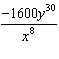3.  Simplify each base using the properties of powers. Then, write all the fractions in the simplest terms and ensure there are no negative exponents.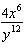4.  When multiplying and the base is the same, add exponents.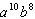5.  Multiply the first number by the second number. Add variable exponents when the base is the same.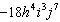6. Multiply the number times itself, then that times itself the number of times of the power outside the braces. Multiply that number times itself the number of times of the power outside the parentheses. When a variable with an exponent has a power, the exponents are multiplied.7.  To divide two powers that have the same base, subtract the exponents.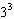8. When a power has a power, multiply the exponents. To divide two powers that have the same base, subtract the exponents.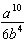9. When the power is negative, invert the fraction, then multiply exponents and subtract bottom exponent from top exponent.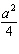10. Invert negative powers. When dividing, subtract the bottom exponent from the top exponent with the same base. If the answer is negative, the result goes on the bottom. If positive, the result goes on top.(source)